Nonparametric NAR-ARCH Modelling of Stock Prices by the Kernel Methodology

Journal of Economics and Financial Analysis, Vol:2, No:2 (2018) 105-120

# Nonparametric NAR-ARCH Modelling of Stock Prices by the Kernel Methodology

## Mohamed CHIKHI*a, Ali BENDOBb

a Department of Commerce, University of Ouargla, Algeria

b Department of Economics, University of Ain-Temouchent, Algeria

## Abstract

This paper analyses cyclical behaviour of Orange stock price listed in French stock exchange over 01/03/2000 to 02/02/2017 by testing the nonlinearities through a class of conditional heteroscedastic nonparametric models. The linearity and Gaussianity assumptions are rejected for Orange Stock returns and informational shocks have transitory effects on returns and volatility. The forecasting results show that Orange stock prices are short-term predictable and nonparametric NAR- ARCH model has better performance over parametric MA-APARCH model for short horizons. Plus, the estimates of this model are also better comparing to the predictions of the random walk model. This finding provides evidence for weak form of inefficiency in Paris stock market with limited rationality, thus it emerges arbitrage opportunities.

Keywords: Final Prediction Error; Kernel; Bandwidth; Conditional Heteroscedastic Functional Autoregressive Process; Orange Stock Price; Forecasts.

JEL Classification: C14, C22, C58, G17.

* Corresponding author. Tel: (+213) 043 79 83 14

E-mail addresses: mchikhi00@gmail.com (M. Chikhi), bendobali4@gmail.com (A. Bendob)

1. ## Introduction

The empirical models in finance have been proposed to predict the share returns (Sharpe, 1964; Ross, 1976) or the premium of financial derivatives (Black and Scholes, 1973). Majority of these models assumes (1) linearity and (2) Gaussianity, and frequently being utilized as an empirical tool in testing validation of these assumptions that opine (1) a linear impact running from past values of stock market returns on to current ones, and (2) an identically and independently distribution (normal distribution) of the residuals.

Stock prices are characterized by a particular structure that is fundamental to addressing the problem of modelling. Thus, the presence of nonlinearities in the financial series has important implications particularly with regard to the weak form of efficiency markets. Indeed, if a series exhibit a nonlinear dynamic, this implies nonlinear dependencies between the observations. In the other words, the past can influence the random variations of today. Theoretically, nonlinearity is the real source of diversity, complexity and volatility (Campbell, Lo, and MacKinlay, 1997).

Financial time series, especially stock returns, is no longer predictable by linear models since these processes are based on restrictive assumptions such as presence of symmetric distribution and a linear structure. The Gaussian linear models can only generate symmetric behaviours in series, and cannot reproduce effects of presence of transaction costs and heterogeneous expectations of agents on stock markets. Moreover, the linear processes do not explain the phenomena of clustering of volatility and structural change.

Many works are interested in the field of parametric nonlinear time series. Such parametric nonlinear models may be too restrictive in many cases. This leads to various nonparametric techniques being used to model nonlinear time series data that may be allowed to speak for themselves. The development of these methods has provided a solid theoretical basis for the nonlinear time series analysis (Robinson, 1983). They are considered as an alternative to the nonlinearity problem. The financial series is generally characterized by a time- varying volatility that can be modelled by nonparametric ARCH-type models, which corresponds to a specific representation of nonlinearity allowing for simplified modelling of uncertainty.

The nonparametric methods have boomed since studies of Tjostheim and Auestad (1994) and Yang and Tschernig (1998). Cheng and Tong (1992) propose a cross validation criterion, whereas Vieu (1995) puts forward penalized cross validation. On contrary, Yang and Tschernig (1998) suggest using the corrected nonparametric FPE criterion by introducing a new term in the FPE criterion adopted by Tjostheim and Auestad (1994). Andersen, Bollerslev, and Diebold (2009) review and provide a unified framework for interpreting the parametric and nonparametric approaches, which include ARCH filters and smoothers designed to measure the volatility over infinitesimally short horizons, as well as recently-popularized realized volatility measures for fixed-length time intervals. Chikhi and Diebolt (2010) test weak form of Paris Stock Exchange market efficiency by nonparametric methods using kernel methodology. Kristensen (2010) proposes a kernel weighted version of the standard realized integrated volatility estimator and shows consistency and asymptotic normality of the kernel smoothed realized and the filtered spot volatilities. Mwamba (2011) tests the predictability of stock prices in more efficient and developed markets (US/UK) using dynamic random walk and univariate nonparametric kernel models based on local linear estimator. The nonparametric final prediction error (FPE) criterion introduced by Auestad and Tjostheim (1994), which is used to determine the appropriate number of lags. But this criterion has highest probability of over estimation. In addition, the nonparametric estimators are consistent under standard conditions used in nonparametric time series. There is a larger asymptotic probability to overfit than to underfit the correct model, where overfitting is meant to always include all correct lags plus some irrelevant lags. Therefore, we add in asymptotic final prediction error (AFPE), a correction factor that depends on the number of lags and observations. Hou and Suardi (2012) study oil price return volatility and show that out-of-sample volatility forecast of the nonparametric GARCH model yields superior performance relative to an extensive class of parametric GARCH models. In turn, Er and Fidan (2013) use the nonparametric GARCH model to analyze volatility of daily stock returns of Istanbul Stock Exchange 100 (ISE 100) market and find plausible results. On the other hand, Kung (2016) employs nonparametric kernel regression to deal with changing volatility based on Nadaraya-Watson estimator and compares the index option prices under nonparametric model with those under Black–Scholes and Stein–Stein models. The Nadaraya-Watson estimator has a poor bias rate if density of the lagged variable is not sufficiently smooth, especially with nonlinear processes. It is just a special case of local linear estimator since it locally estimates a function that is constant. The asymptotic bias of the Nadaraya-Watson estimator is more complicated than the asymptotic bias of local linear estimator. This estimator needs only continuity of the density to have an optimal convergence rate (Hardle, Tsybakov, and Yang, 1998).

The present article, in contrast to studies that use Nadaraya-Watson estimator and FPE criterion, employs corrected asymptotic final prediction error (CAFPE) based on local linear estimator to estimate nonparametrically conditional mean and conditional volatility by selecting optimal bandwidth and lags. We apply this technique to use examine informational shocks and nonlinearities in Orange stock returns using nonparametric version of conditional heteroscedastic nonlinear models. The short-term predictability of price of Orange Company at Paris stock markets provides evidence for inefficiency of Paris stock market (in a weak level) with limited rationality, which emerges arbitrage opportunities.

The remaining paper is structed as following. Next section presents descriptive analysis of daily Orange stock price returns and volatility. In third section, we present core results of nonparametric modelling of stock Orange return series wehre we compare predictive quality of conditional heteroscedastic nonparametric Autoregressive (NAR-ARCH) and parametric MA-APARCH models with that of a random walk. The last section concludes the study outlining core findings.

2. ## Descriptive Statistics and Data characteristics

The data considered in this paper consists of daily price of Orange share listed in France stock exchange. The data of 17 years of daily price downloaded from Yahoo Finance covering a historical period from January 3, 2000 to February 2, 2017, (4449 observations). Unit root tests results (Philips and Perron, 1988; Elliott, Rothenberg and Stock, 1996; Ng and Perron, 2001) reported in table 1 show that the series is characterized by the presence of unit roots. The series is finally differenced by calculating returns as rt=100*ln(ORAt/ORAt-1), where ORAt is value of the index (Orange) at time point t (see figure 1).

Table 1. Unit root tests

SeriesPhilips- PerronElliott- Rothenberg-StockNg-Perron
MZaMZtMSBMPT
Logarithmic series-1.4070
(-1.9400)
0.0760
(3.2600)
-0.1810
(-8.1000)
-0.18000
(-1.9800)
0.9940
(0.2330)
52.9490
(3.1700)
Returns-62.7870
(-1.9400)
53.5330
(3.2600)
-366.018
(-8.1000)
-13.5200
(-1.9800)
0.0369
(0.2330)
0.0752
(3.1700)

Notes: The numbers in the table are asymptotic critical values of unit root tests (the null hypothesis is H0: series has unit root) with Bartlett kernel spectral estimation method of Andrews bandwidth. The tested equation uses unrestricted intercept without any kinds of trends. The numbers in parentheses are asymptotic critical values at 5% significance level. MZa, MZt, MSB, MPT are the Ng-Perron statistics. Tested model uses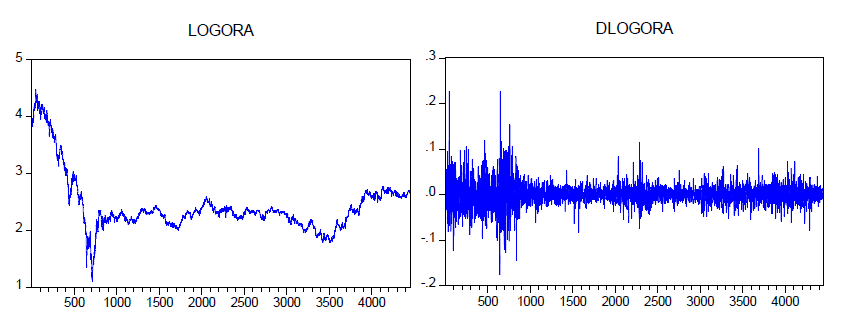Figure 1. Stock exchange Orange (logarithmic and returns)

In table 2, we provide some descriptive statistics for the return series. As it is seen from the skewness and kurtosis values, Orange return series is positively skewed and leptokurtic. The right tail of distribution is longer than the left one. That is, negative return frequencies occur much more than positive returns (see also Figure 2-A). The observed asymmetry may indicate the presence of nonlinearities in the evolution process of returns. The scatter plot of the series (Figure 2-B) does not appear in the form of a regular ellipsoid and confirms nonlinearity. Furthermore, the distribution is leptokurtic. The series is characterized by the presence of ARCH effects (see table 2).

Table 2. Main characteristics of Orange returns series

 Mean Median StandardDeviation Skewness Kurtosis Jarque-Bera ARCH(1) N -0.0293 0.0000 2.4002 0.4574 12.0127 15209.64(0.0000) (0.0000) 4448

Notes: The numbers in the table are statistics of descriptive analysis with the null hypothesis of H0: there is no difference between our distribution and a normal distribution. The numbers in the paranthesis are critical probabilities.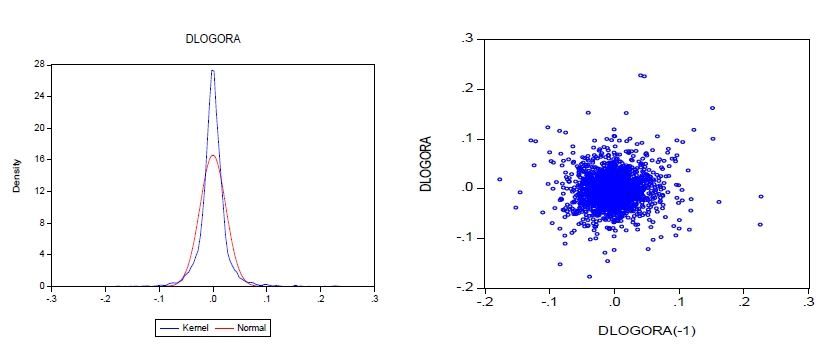(A): Kernel estimation of density (B): Scatter plot of Orange variations

Figure 2. Kernel Estimation of Density and Scatter Plot of Orange Variations

Table 3 shows results of BSD test (Brock, Dechert, Scheinkman & LeBaron, 1996) that analyzes presence of nonlinear short-term dependencies. According to the results, the test rejects assumption of random walk as its statistics is greater than the critical value at 5% significance level. This indicates that the stock exchange Orange prices are short-term predictable.

Table 3. BDS test results on the series on Orange stock returns

 m BDS stat. m BDS stat. 2 20.541 7 41.574 3 26.459 8 45.353 4 30.934 9 49.637 5 34.563 10 54.442 6 37.986

Notes: The numbers in the table are BDS test statistics with the null hypothesis of H0: Returns are Independent and Identically Distributed (i.i.d). The BDS statistics are calculated by the fraction of pair’s method of epsilon with value of 0.7, and maximum correlation dimension (m) of 10. The critical values are 1.645, 1.960, and 2.575 for the 10%, 5%, and 1% significance levels respectively.

We have to analyse the cyclical behaviour of returns by considering longer horizons. So, we estimate fractional integration coefficient by the GPH method (Geweke and Porter-Hudak, 1983) with different spectral bandwidths. From Table 4, it's obvious that the stock exchange Orange return series is not generated by long memory process. The values of the Student statistic (with a power of 0.8) are strictly less than the critical value at 5%. The returns are short-term predictable: the presence of a short memory indicates that agents cannot anticipate their returns to a sufficiently long horizon. Indeed, the observed movements appear as the result of transitory exogenous shocks.

Table 4. Results from the ARFIMA estimation using spectral methods on Orange returns

 Bandwidth d Student stat. Prob. Without bandwidth 0.035 1.553 0.120 Rectangular 0.006 0.273 0.784 Bartlett 0.012 0.869 0.384 Daniell 0.011 0.673 0.500 Tukey 0.010 0.669 0.503 Parzen 0.015 1.154 0.248 B-priest 0.008 0.418 0.675

Notes: The numbers in the table are the estimated fractional integration coefficient and its t-statistic using different spectral bandwidths (the null hypothesis H0: d is not significant). The numbers in the last column are critical probabilities.

The results of the Hinich bispectrum test (Hinich and Patterson, 1989) for linearity and Gaussianity are shown in Table 5. The Gaussianity statistic is strictly greater than the critical value of standard normal at 5%, and the linearity statistic is greater than the critical value of 2 at 5% with two degrees of freedom. We reject the null hypothesis of linearity and Gaussianity.

Table 5. Hinich bispectrum test for linearity and Gaussianity

 Frame Size Lattice Points Test Quantile Linearity Gaussianity 67 272 0.8 31.409(0.000) 7978.107(0.000)

Notes: The numbers in the table are nonparametric Hinich bispectral test statistics with the null hypothesis (H0) of linearity and Gaussianity, obtaining the chi-squared statistic for testing the significance of individual bispectrum estimates by exploiting its asymptotic distribution. The numbers in the parenthesis are critical probabilities.

3. ## Nonparametric Modelling

The modelling of our series turns towards a nonlinear process but its form is unknown. Faced with this situation, we use the nonparametric version of the conditional heteroscedastic nonlinear autoregressive process based on local linear kernel estimator (Tschernig and Yang, 2000). Any nonparametric model fitting requires the selection of the relevant Markov coefficients and bandwidths. For that we use the nonparametric Corrected Asymptotic Final Prediction Error (CAFPE) introduced by Yang and Tschernig (1998):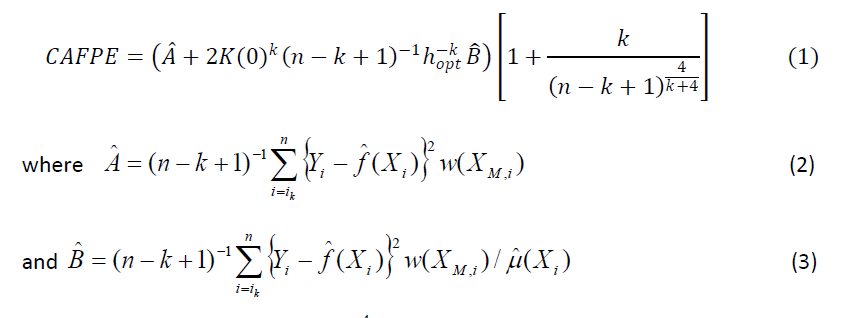The factor 1 + k/(n - k + 1)4/k+4 penalizes the over-estimation of Markov coefficients. We can use here bandwidths of the order (n - k + 1)-1/(k+4)

for estimating fˆ, μˆ( Xi) is the kernel density estimator and hopt is the optimal bandwidth (Tschernig and Yang, 2000, p. 462). A is estimated using this optimal bandwidth hopt and Bˆ using any bandwidth of order (n - k + 1)-1/(k+4). The weight function w(.) is specified by: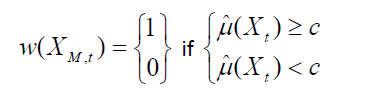where the constant c is determined such that observations are excluded and denotes the next larger integer of 0.05(n - k + 1).

In all cases, the maximum number of lags is restricted to be four and the largest lag to be considered is eight. However, the idea introduced by Tjostheim and Auestad (1994) is to choose the Markov coefficients with respect to their contribution to this reduction. Thus, a bandwidth must be specified for each computation of the CAFPE. This can be done either by estimating the optimal bandwidth hopt or by conducting a grid search. To compute the residuals and the density that are used to estimate the expected variance of the conditional mean, we use a Silverman’s bandwidth (Silverman, 1986, eq. (4.14), p. 86). Moreover, the grid search procedure for determining the optimal bandwidth is used for all CAFPE where the grid covers the interval [0,2hs,2hs] in 24 steps and in order to estimate the Orange stock exchange series, we use the Gaussian kernel (Chikhi and Diebolt, 2010).

Table 6 reports the results of the identification of the Orange stock exchange return process. It contains selected number of lags, values of the criterion, estimated optimal bandwidth, and estimated variance of the white noise. According the test results, estimated criterion is equal to 0.00051 and selected Markov coefficients are: 1, 3, 5 and 8. We notice that the residuals (Figure 3-A) are characterized by asymmetric and leptokurtic distribution (Figure 3-B and Table 6).

Table 6. Nonparametric specification and estimation of conditional mean using the local linear estimator

 Nonparametric lag selection Nonparametric estimation of conditional mean Lag selection 1, 3, 5, 8 The user-specified bandwidth 0.0231 Optimal bandwidth hn 0.0231 The estimated criterionCAFPE 0.00051 Associated criterion value CAFPE 0.00044 Estimated error variance 0.000463 Estimated variance ofwhite noise ˆ 2 0.000463 Estimated conditional error variance 0.00039 Skewness Kurtosis Jarque-Bera ARCH(1) 0.457 12.012 15209.64(0.0000) (0.0000)

Notes: The numbers in the upper part of the table are selected number of lags, values of the criterion, estimated optimal bandwidth, and estimated variance of the white noise. The numbers in the bottom part of the table are descriptive analysis and ARCH-LM statistic of NAR residuals (the null hypothesis H0: there is no difference between our distribution and a normal distribution and there is no ARCH effect in the return series). The numbers in the parenthesis are critical probabilities.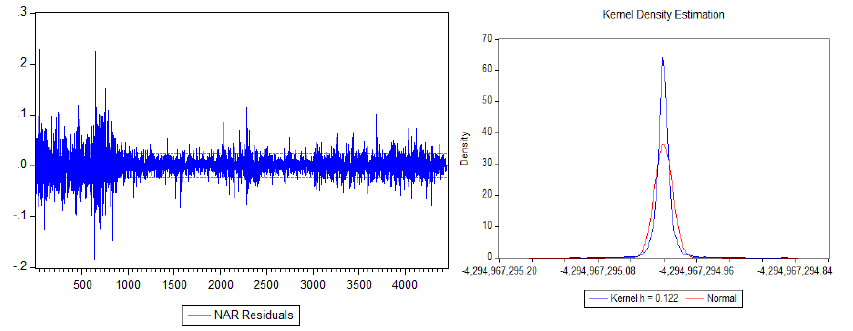(A): Nonparametric NAR residual using lags 3 & 8 (B): Kernel estimator of residuals’ density

Figure 3. Nonparametric NAR residual using lags 3 and 8 & Kernel estimator of residuals’ density

Table 7 clearly rejects the white noise hypothesis indicating that these residuals could present a nonlinear dependence structure as the BDS statistics lead us to rejection of i.i.d hypothesis for all embedding dimensions. However, the residuals still can be modelled using the ARCH models (Table 6) because the presence of an ARCH effect is confirmed by the result of the ARCH-LM test on the residuals ( nR 2 13.904 2 (1) ). Consequently, we identify and estimate the conditional volatility by the kernel methodology.

Table 7. BDS test on NAR and NAR-ARCH residuals

 m NAR residualBDS statistics m NAR-ARCH residualBDS statistics 2 10.243 2 0.114 3 15.574 3 0.741 4 21.547 4 0.015 5 28.279 5 1.247 6 39.412 6 1.452 7 45.254 7 1.694 8 50.247 8 1.781 9 57.791 2 0.114 10 62.432 3 0.741

Notes: The numbers in the table are BDS statistics on NAR and NAR-ARCH residuals (the null hypothesis H0: Returns are Independent and Identically Distributed (i.i.d)) that are calculated by the fraction of pair’s method of epsilon with value of 0.7.

Table 8 presents estimation results for identification of conditional volatility. As in table 6, we display estimates for lag selections, criterion values, optinal bandwidth estimation, and white noise variance estimation. The estimated criterion is equal to 0.0000014 and selected Markov coefficients are: 2, 3, 7 and 8. We can easily notice that the nonparametric NAR-ARCH residuals (Figure 4-A) are a white noise and have no serial correlation (Table 7). In addition, there are signs of nonlinearity (Figure 4-B) where the series has an asymmetric dynamic behaviour. These nonlinear dependencies of conditional mean and conditional variance can reproduce effects of transaction costs variable and heterogeneous expectations of agents on the stock market.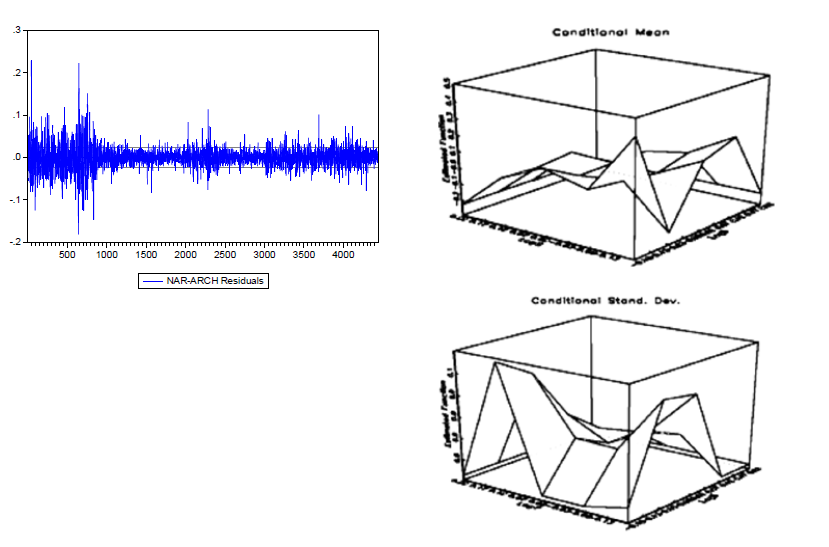(A): Nonparametric NAR-ARCH residuals using lags 3 and 7 (B): Conditional mean and variance functions

Figure 4. Nonparametric NAR-ARCH residuals using lags 3 and 7 & Conditional mean and variance functions

Table 8. Nonparametric specification and estimation of conditional volatility using the local linear estimator

 Nonparametric lag selection Nonparametric estimation of conditional volatility Lag selection 2,3,7,8 The user-specified bandwidth 0.0214 Optimal bandwidth hn 0.0214 The estimated criterionCAFPE 0.0000014 Associated criterion value CAFPE 0.0000012 Estimated error variance 0.0000012 Estimated variance ofwhite noise ˆ 2 0.0000012 Estimated conditional error variance 0.0000010

Notes: The table shows selected number of lags, values of the criterion, estimated optimal bandwidth, and estimated variance of the white noise.

To compare forecasting performance of nonparametric NAR-ARCH, MA- APARCH, and the random walk models, we use the root mean square error criterion (RMSE). For the in-sample predictions, the estimation of the nonparametric NAR-ARCH model is only based on 4418 observations, in order to make further comparisons with the predictions of the 30 remaining observations.

Table 9 contains statistical comparisons of forecasts provided by mentioned three models. We find that both nonparametric NAR-ARCH and MA-APARCH models outperform the random walk model in all forecasting time horizons. The NAR-ARCH model tend to have better predictive results comparing to MA- APARCH one in 1, 2, 30, and 90 days for in-of-sample predictions, as well as in 1, 2, 15, and 90 days for out-of-sample predictions. MA-APARCH outperforms NAR- ARCH only in 15 days for in-of-sample predictions and in 30 days for out-of- sample predictions. Morover, values of RMSE criterion increase with the prediction horizons because all the models take into account only the short-term memory of the series, and therefore completely neglect the long-term memory. In other words, the predictive power for Orange stock returns doesn’t reflect the possibility to forecast up to the longest horizon.

Table 11. Comparison of predictive qualities using the root mean absolute error criterion (RMSE)

 Prediction Horizon Bandwidth used for nonparametricprediction Nonparametric NAR-ARCH MA(2)- APARCH Random Walk 1 0.0231 0.00014 0.00028 0.0198 in-sample predictions 2 0.0230 0.00309 0.00314 0.0219 15 0.0226 0.00531 0.00529 0.0860 30 0.0221 0.00573 0.00607 0.1115 90 0.0215 0.00820 0.00891 0.1784 1 0.0471 0.00044 0.00095 0.0240 out-of-sample predictions 2 0.0470 0.00298 0.00317 0.0339 15 0.0466 0.00609 0.00618 0.0930 30 0.0461 0.00650 0.00646 0.1317 90 0.0458 0.00902 0.00911 0.1947

Notes: The numbers in the table are the root mean absolute error criterion (RMSE).

Given that the Orange returns are characterized by the presence of nonlinear dynamics in the equations of the mean and conditional variance and by heteroscedasticity, the nonparametric modelling allows computation of better short-term forecasts than the random walk model. This model is clearly superior to random walk model. Indeed, the returns are short-term predictable: the absence of a long memory indicates that agents can only anticipate their returns to a short time horizon. Indeed, the observed movements appear as the result of transitory exogenous shocks.

4. ## Concluding Remarks

This paper examines predictability of stock share prices using nonparametric nonlinear autoregressive model with nonparametric ARCH errors. This study is limited only to stock Orange price indices. In this context, we implement local linear kernel method to estimate our nonparametric model, taking into account the nonlinear phenomenon in the conditional mean and variance. According to our analysis, we document that informational shocks have transitory consequences on stock returns and volatility. Specifically, forecasts of nonlinear parametric and nonparametric models show a clear improvement comparing to the random walk model at all horizons. Especially, in nonparametric NAR-ARCH model outperforms random walk model in all 1-90 days in- and out-sample predictions. In addition, estimations of root mean square error suggest that forecasts generated by nonparametric approaches tend to have relatively close (similar) estimates with more accurance.

The empirical method used for the determination of Markov coefficients is not based on any validation theorem. Generally, existing results admit implicitly that the observed process is k-Markovian. This hypothesis is difficult to verify in practice. Despite this difficulty, the nonparametric methods are still a powerful tool for studying time series. If the structure of nonlinear parametric process is unknown, we could, through the nonparametric modelling, try to reduce our ignorance and minimize the random effects in the dynamic systems. Our results provide evidence for inefficiency of Paris stock market in a weak level with limited rationality, which emerges arbitrage opportunities.

## References

Andersen, T.G., Bollerslev, T., and Diebold, F.X. (2009). Parametric and nonparametric measurement of volatility. In Ait-Sahalia Y. and Hansen L.P. (eds.), Handbook of Financial Econometrics. Press: North Holland.

Black, F., and Scholes, M. (1973). The Pricing of Options and Corporate Liabilities.Journal of Political Economy, 81(3), 637-654.

Brock, W.A., Dechert, W.D., Scheinkman, J.A., and LeBaron, B., A. (1996). Test for independence Based on the Correlation Dimension. Econometric Reviews, 15, 197-235.

Campbell, J.Y., Lo, A., and MacKinlay, A.C. (1997). The Econometrics of Financial Markets. Princeton University Press, Princeton, N.J.

Cheng, B., and Tong, H. (1992). On consistent nonparametric order determination and chaos. Journal of the Royal Statistical Society, Series B, 54, 427-449.

Chikhi, M., and Diebolt, C. (2010). Nonparametric Analysis of Financial Time Series by the Kernel Methodology. Quality & Quantity. International Journal of Methodology (Springer), 44, 865-880.

Elliot, G., Rothenberg, T.J., and Stock, J.H. (1996). Efficient Tests for an Autoregressive Unit root. Econometrica, 64, 813-836.

Er, S., and Fidan, N. (2013). Modelling Istanbul Stock Exchange-100 Daily Stock Returns: A nonparametic GARCH Approach. Journal of Business, Economics & Finance, 2 (1), 36-50.

Geweke, J., and Porter-Hudak, S. (1983). The Estimation and Application of Long Memory Time Series Models. Journal of Time Series Analysis, 4, 221-238.

Hardle, W., Tsybakov, A.B., and Yang, L. (1998). Nonparametric vector autoregression. Journal of Statistical Planning and Inference, 68, 221-245.

Hinich, M.J., and Patterson, D.M. (1989). Evidence of nonlinearity in the trade-by- trade stock market return generating process. In W.A. Barnett, J. Geweke and K. Shell (eds.), Economic Complexity: Chaos, Sunspots, Bubbles and Nonlinearity International Symposium in Economic Theory and Econometrics, 383-409. Cambridge: Cambridge University Press.

Hou, A., and Suardi, S. (2012). A Nonparametric GARCH model of Crude Oil Price Return Volatility. Energy Economics, 34(2), 618-626.

Kristensen, D. (2010). Nonparametric filtering of Realized Spot Volatility: A Kernel- Based approach. Econometric Theory, 26 (1), 60-93.

Kung, J.J. (2016). A nonparametric kernel regression approach for pricing options on stock market index. Journal of Applied Economics, 48(10), 902-913.

Mwamba, J.M. (2011). Modelling stock price behaviour: The Kernel approach. Journal of Economics and International Finance, 3(7), 418-423.

Ng, S., and Perron, P. (2001). Lag Length Selection and the Construction of Unit Root Tests with Good Size and Power. Econometrica, 69(6), 1519–1554.

Phillips, P.C.B., and Perron, P. (1988). Testing for a Unit Root in Time Series Regression. Biometrika, 75 (2): 335–346.

Robinson, P.M. (1983). Nonparametric estimators for time series. Journal of Time Series Analysis, 4, 185-207.

Ross, S.A. (1976). The arbitrage theory of capital asset pricing. Journal of Economic theory, 13(3), 341-360.

Sharpe, W.F. (1964). Capital Asset Prices: A Theory of Market Equilibrium under Conditions of Risk. Journal of Finance, 19(3), pp. 425-442.

Silverman, B.W. (1986). Density estimation for Statistics and data analysis. Chapman & Hall.

Tjostheim, D., and Auestad, B. (1994). Nonparametric identification of nonlinear time series: selecting significant lags. Journal of American Statistical Association, 89, 1410-1419.

Tschernig, R., and Yang, L. (2000). Nonparametric Lag Selection for Time Series. Journal of Time Series Analysis, 21(4), 457-487.

Vieu, P. (1995). Order choice in nonlinear autoregressive models. Statistics, OPA, 26, 307-328.

Yang, L., and Tschernig, R. (1998). Non- and Semiparametric Identification of Seasonal Nonlinear Autoregression Models. SFB 373 Discussion Papers 1998, 114. Humboldt University of Berlin, Interdisciplinary Research Project 373, Quantification and Simulation of Economic Processes.